Next: Proof of Theorem 5 Up: Introduction to Number Theory Previous: Powers modulo a prime   Contents

## Primitive roots

Recall that theorem 5 says that if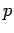is a prime, there is an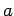such that the equation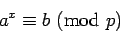has a solution for any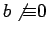. Such anis called a primitive root of, and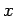is called the discrete logarithm of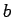.

We showed in the beginning of section 2 that it is easy to obtaingivenand. Findinggivenandis much harder. Many modern encryption systems are based on the fact that no efficient way of computing discrete logarithms is known.

Lemma 10   Let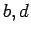be as in Lemma 9. If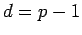, thenis a primitive root.

Proof: If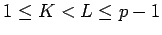and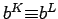, then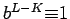. Since this can't happen by assumption, the firstpowers ofmust all be different, hence must include all numbers between 1 and. height 8pt width 4pt

It is often possible to find a primitive root ifhas a small number of prime divisors. We will use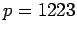as an example.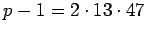. By Lemmas 10 and 9, ifis not a primitive root, then we will either have,, or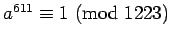.and 3 fail, but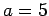satisfies all three conditions, so it is a primitive root. (we could tell that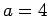would not be a primitive root without testing. Why?)

It is easy to show that, ifis a primitive root,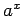is a primitive root if and only if. In this example, this means the number of primitive roots is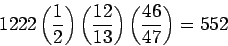Thus, if we had just chosenat random, the probability that it would be a primitive root is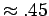. Choosingat random and testing until we found a primitive root would not be expected to take too long.

This is an example of a probabilistic algorithm. It is possible for it to take a long time, but the amount of time needed on average is reasonably small. We will see many other probabilistic algorithms later.Next: Proof of Theorem 5 Up: Introduction to Number Theory Previous: Powers modulo a prime   Contents
Translated from LaTeX by Scott Sutherland
2002-12-14